1. /
2. CBSE
3. /
4. Class 10
5. /
6. Sample paper for class...

# Sample paper for class 10 Maths 2018-19

Download CBSE sample paper for class 10 Maths for board exams available for download in myCBSEguide mobile app. The best app for CBSE students now provides class 10 maths sample paper includes all questions from Mathematics – Textbook for class X – NCERT Publication, Guidelines for Mathematics Laboratory in Schools, class X – CBSE Publication, Laboratory Manual – Mathematics, secondary stage – NCERT Publication, Mathematics exemplar problems for class X, NCERT Publication. In the session 2018-19, CBSE will conduct board exam for all CBSE class 10 students which will cover the whole book.

For study on the go download myCBSEguide app for android phones. Sample paper for class 10 Maths and other subjects are available for download as PDF in app too.

## Class 10 Mathematics Sample papers

General Instructions:

1. All questions are compulsory.
2. The question paper consists of 30 questions divided into four sections A, B, C and D.
3. Section A contains 6 questions of 1 mark each. Section B contains 6 questions of 2 marks each. Section C contains 10 questions of 3 marks each. Section D contains 8 questions of 4 marks each.
4. There is no overall choice. However, an internal choice has been provided in four questions of 3 marks each and three questions of 4 marks each. You have to attempt only one of the alternatives in all such questions.
5. Use of calculators is not permitted.

### Section A Question numbers 1 to 6 carry 1 mark each

1. Write whether the rational number {tex}\frac{7}{{75}}{/tex} will have a terminating decimal expansion or a nor-terminating repeating decimal expansion.
Ans. Non terminating repeating decimal expansion.

2. Find the value(s) of k, if the quadratic equation {tex}3{x^2} – k\sqrt 3 x + 4 = 0{/tex} has equal roots.
Ans. {tex}k = \pm 4{/tex}

3. Find the eleventh term from the last term of the AP:
27, 23, 19, …, –65.
Ans. {tex}{a_{11}} = – 25{/tex}
4. Find the coordinates of the point on y-axis which is nearest to the point (–2, 5).
Ans. {tex}\left( {0,\,5} \right){/tex}
5. In given figure, {tex}ST||RQ,{\text{ }}PS = 3\,cm{/tex} and {tex}SR = 4{\text{ }}cm.{/tex} Find the ratio of the area of {tex}\Delta PST{/tex} to the area of {tex}\Delta PRQ.{/tex}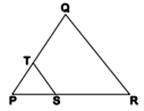Ans. {tex}9:\,49{/tex}
6. If {tex}cos{\text{ }}A = \frac{2}{5},{/tex} find the value of {tex}4 + 4\,ta{n^2}A{/tex}
Ans. 25

### Section B Question numbers 7 to 12 carry 2 marks each.

7. If two positive integers p and q are written as {tex}p = {a^2}{b^3}{\text{ }}and{\text{ }}q = {a^3}{b};{\text{ }}a,\;b{/tex} are prime numbers, then verify:
{tex}LCM\left( {p,q} \right) \times HCF\left( {p,q} \right) = pq{/tex}

Ans. {tex}LCM(p,\,q) = {a^3}{b^3}{/tex}
{tex}HCF(p,\,q) = {a^2}b{/tex}
{tex}LCM\left( {p,q} \right) \times HCF\left( {p,q} \right) {/tex}{tex} = {a^5}{b^4} = \left( {{a^2}{b^3}} \right)\left( {{a^3}b} \right) = pq{/tex}
8. The sum of first n terms of an AP is given by {tex}{S_n} = 2{n^2} + 3n.{/tex} Find the sixteenth term of the AP.

Ans. Sn = 2n2 + 3n

S1 = 5 = a1
S2 = a1 + a2 = 14 {tex} \Rightarrow {/tex} a2 = 9
d = a2 – a1 = 4
a16 = a1 + 15d = 5 + 15(4) = 65
9. Find the value(s) of k for which the pair of linear equations {tex}kx + y = {k^2}{\text{ }}and{\text{ }}x + ky = 1{/tex} have infinitely many solutions.

Ans. For pair of equations kx + 1y = k2 and 1x + ky = 1
We have: {tex}\frac{{{a_1}}}{{{a_2}}} = \frac{k}{1},\,\frac{{{b_1}}}{{{b_2}}} = \frac{1}{k},\,\frac{{{c_1}}}{{{c_2}}} = \frac{{{k^2}}}{1}{/tex}
For infinitely many solutions, {tex}\frac{{{a_1}}}{{{a_2}}} = \frac{{{b_1}}}{{{b_2}}} = \frac{{{c_1}}}{{{c_2}}}{/tex}
{tex}\therefore \frac{k}{1} = \frac{1}{k} \Rightarrow {k^2} = 1 \Rightarrow k = 1,\, – 1\,…(i){/tex}
{tex}and\,\frac{1}{k} = \frac{{{k^2}}}{1} \Rightarrow {k^3} = 1 \Rightarrow k = 1\,…(ii){/tex}
From (i) and (ii), k = 1
10. If {tex}\left( {1,\,\frac{p}{3}} \right){/tex} is the mid-point of the line segment joining the points (2, 0) and {tex}\left( {0,\,\frac{2}{9}} \right),{/tex} then show that the line {tex}5x + 3y + 2 = 0{/tex} passes through the point {tex}\left( { – 1,{\text{ }}3p} \right).{/tex}

Ans. Since {tex}\left( {1,\,\frac{p}{3}} \right){/tex} is the mid-point of the line segment joining the points {tex}\left( {2,\,0} \right)\,and\,\left( {0,\,\frac{2}{9}} \right){/tex} therefore, {tex}\frac{p}{3} = \frac{{0 + \frac{2}{9}}}{2} \Rightarrow p = \frac{1}{3}{/tex}
The line {tex}5x + 3y + 2 = 0{/tex} passes through the point {tex}\left( {-1,{\text{ }}1} \right){\text{ }}as{\text{ }}5\left( {-1} \right) + 3\left( 1 \right) + 2 = 0{/tex}
11. A box contains cards numbered 11 to 123. A card is drawn at random from the box. Find the probability that the number on the drawn card is
(i) a square number
(ii) a multiple of 7

Ans. (i) P(square number){tex} = \frac{8}{{113}}{/tex}
(ii) P(multiple of 7){tex} = \frac{{16}}{{113}}{/tex}
12. A box contains 12 balls of which some are red in colour. If 6 more red balls are put in the box and a ball is drawn at random, the probability of drawing a red ball doubles than what it was before. Find the number of red balls in the bag.

Ans. Let number of red balls be = x
{tex}\therefore P(red\,ball) = \frac{x}{{12}}{/tex}
If 6 more red balls are added:
The number of red balls = x + 6
{tex}P(red\,ball) = \frac{{x + 6}}{{18}}{/tex}
Since, {tex}\frac{{x + 6}}{{18}} = 2\left( {\frac{x}{{12}}} \right) \Rightarrow x = 3{/tex}
{tex}\therefore {/tex} There are 3 red balls in the bag.

### Section C Question numbers 13 to 22 carry 3 marks each.

13. Show that exactly one of the numbers {tex}n,{\text{ }}n + 2{\text{ }}or{\text{ }}n + 4{/tex} is divisible by 3.

Ans. Let n = 3k, 3k + 1 or 3k + 2.
(i) When n = 3k:
n is divisible by 3.
n + 2 = 3k + 2 {tex} \Rightarrow {/tex} n + 2 is not divisible by 3.
n + 4 = 3k + 4 = 3(k + 1) + 1 {tex} \Rightarrow {/tex} n + 4 is not divisible by 3.

(ii) When n = 3k + 1:
n is not divisible by 3.
n + 2 = (3k + 1) + 2 = 3k + 3 = 3(k + 1) {tex} \Rightarrow {/tex} n + 2 is divisible by 3.
n + 4 = (3k + 1) + 4 = 3k + 5 = 3(k + 1) + 2 {tex} \Rightarrow {/tex} n + 4 is not divisible by 3.

(iii) When n = 3k + 2:
n is not divisible by 3.
n + 2 = (3k + 2) + 2 = 3k + 4 = 3(k + 1) + 1 {tex} \Rightarrow {/tex} n + 2 is not divisible by 3.
n + 4 = (3k + 2) + 4 = 3k + 6 = 3(k + 2) {tex} \Rightarrow {/tex} n + 4 is divisible by 3.
Hence exactly one of the numbers n, n + 2 or n + 4 is divisible by 3.
14. Find all the zeroes of the polynomial {tex}3{x^4}{\text{ + }}6{x^3} – 2{x^2} – 10x – 5{/tex} if two of its zeroes are {tex}\sqrt {\frac{5}{3}} \,and\, – \sqrt {\frac{5}{3}} .{/tex}

Ans. Since {tex}\sqrt {\frac{5}{3}} \,and\, – \sqrt {\frac{5}{3}} {/tex} are the two zeroes therefore, {tex}\left( {x – \sqrt {\frac{5}{3}} } \right)\left( {x + \sqrt {\frac{5}{3}} } \right) = \frac{1}{3}\left( {3{x^2} – 5} \right){/tex} is a factor of given polynomial.

We divide the given polynomial by {tex}3{x^2} – 5.{/tex}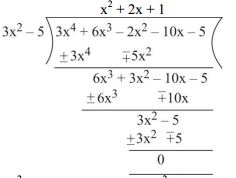For other zeroes, {tex}{x^2} + 2x + 1 = 0 \Rightarrow {(x + 1)^2} = 0,{/tex} {tex}\,x = – 1, – 1{/tex}

{tex}\because {/tex} Zeroes of the given polynomial are {tex}\sqrt {\frac{5}{3}} , – \sqrt {\frac{5}{3}} , – 1\,and\, – 1.{/tex}

15. Seven times a two digit number is equal to four times the number obtained by reversing the order of its digits. If the difference of the digits is 3, determine the number.

Ans. Let the ten’s and the units digit be y and x respectively.
So, the number is 10y + x.
The number when digits are reversed is 10x + y.
Now, 7(10y + x) = 4(10x + y) {tex} \Rightarrow {/tex} 2y = x …(i)
Also x – y = 3 …(ii)
Solving (1) and (2), we get y = 3 and x = 6.
Hence the number is 36

16. In what ratio does the x-axis divide the line segment joining the points {tex}\left( {-4,\;-6} \right){\text{ }}and{\text{ }}\left( {-1,{\text{ }}7} \right)?{/tex} Find the co-ordinates of the point of division.
OR
The points {tex}A\left( {4,-2} \right),B\left( {7,2} \right),C\left( {0,9} \right){\text{ }}and\,D\left( {-3,5} \right){/tex} form a parallelogram. Find the length of the altitude of the parallelogram on the base AB.

Ans. Let x-axis divides the line segment joining {tex}\left( {-4,-6} \right){\text{ }}and{\text{ }}\left( {-1,7} \right){/tex} at the point P in the ratio 1 : k.
Now, coordinates of point of division {tex}P\left( {\frac{{ – 1 – 4k}}{{k + 1}},\frac{{7 – 6k}}{{k + 1}}} \right){/tex}
Since P lies on x-axis, therefore {tex}\frac{{7 – 6k}}{{k + 1}} = 0{/tex}
{tex} \Rightarrow 7 – 6k = 0{/tex}
{tex} \Rightarrow k = \frac{7}{6}{/tex}
Hence the ratio is {tex}1:\,\frac{7}{6} = 6:7{/tex}
Now, the coordinates of P are {tex}\left( {\frac{{ – 34}}{{13}},0} \right).{/tex}
OR
Let the height of parallelogram taking AB as base be h.

Now AB {tex} = \sqrt {{{\left( {7 – 4} \right)}^2} + {{\left( {2 + 2} \right)}^2}} = \sqrt {{3^2} + {4^2}} = 5\,units.{/tex}
{tex}Area\left( {\Delta ABC} \right) = \frac{1}{2}{/tex}{tex}\left[ {4\left( {2 – 9} \right) + 7\left( {9 + 2} \right) + 0\left( { – 2 – 2} \right)} \right]{/tex}{tex} = \frac{{49}}{2}sq\,units.{/tex}
{tex}Now,\,\frac{1}{2} \times AB \times h = \frac{{49}}{2}{/tex}
{tex} \Rightarrow \frac{1}{2} \times 5 \times h = \frac{{49}}{2}{/tex}
{tex} \Rightarrow h = \frac{{49}}{5} = 9.8\,units.{/tex}

17. In given figure {tex}\angle 1 = \angle 2\,and\,\Delta NSQ \cong \Delta MTR,{/tex} then prove that {tex}\Delta PTS \sim \Delta PRQ{/tex}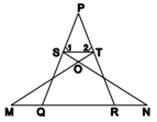OR
In an equilateral triangle ABC, D is a point on the side BC such that {tex}BD = \frac{1}{3}BC.{/tex} Prove that {tex}9A{D^2} = 7A{B^2}.{/tex}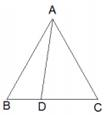Ans. {tex}\angle {\text{SQN = }}\angle {\text{TRM}}{/tex}{tex}{\text{(CPCT as }}\Delta {\text{NSQ}} \cong \Delta {\text{MTR)}}{/tex}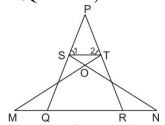Since, {tex}\angle P + \angle 1 + \angle 2 = \angle P + \angle PQR + \angle PRQ{/tex}{tex}\left( {Angle\,sum\,property} \right){/tex}
{tex} \Rightarrow \angle 1 + \angle 2 = \angle PQR + \angle PRQ{/tex}
{tex} \Rightarrow 2\angle 1 = 2\angle PQR{/tex}{tex}(as\,\angle 1 = \angle 2\,and\,\angle PQR = \angle PRQ){/tex}
{tex}\angle 1 = \angle PQR{/tex}
Also {tex}\angle 2 = \angle PRQ{/tex}
And {tex}\angle SPT = \angle QPR(common){/tex}
{tex}\Delta PTS \sim \Delta PRQ{/tex}{tex}(By\,AAA\,similarity\,criterion){/tex}

OR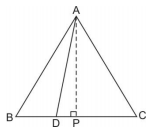Construction: Draw {tex}AP \bot BC{/tex}
{tex}In\,\Delta ADP,\,A{D^2} = A{P^2} + D{P^2}{/tex}
{tex}A{D^2} = A{P^2} + {\left( {BP – BD} \right)^2}{/tex}
{tex}A{D^2} = A{P^2} + B{P^2} + B{D^2} – 2\left( {BP} \right)\left( {BD} \right){/tex}
{tex}A{D^2} = A{B^2} + {\left( {\frac{1}{3}BC} \right)^2} – 2\left( {\frac{{BC}}{2}} \right)\left( {\frac{{BC}}{3}} \right){/tex}
{tex}A{D^2} = \frac{7}{9}A{B^2}\left( {\because BC = AB} \right){/tex}
{tex}9A{D^2} = 7A{B^2}{/tex}

18. In given figure {tex}XY{\text{ }}and\;X’Y'{/tex} are two parallel tangents to a circle with centre O and another tangent AB with point of contact C intersecting {tex}XY{/tex} at A and {tex}X’Y'{/tex} at B. Prove that {tex}\angle AOB = 90^\circ .{/tex}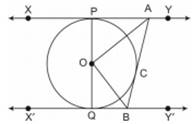Ans. Join OC
In {tex}\Delta OPA{\text{ }}and{\text{ }}\Delta OCA{/tex}
OP = OC (radii of same circle)
PA = CA (length of two tangents)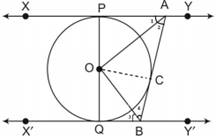AO = AO (Common)
{tex}\therefore \,\Delta OPA \cong \Delta OCA{/tex} (By SSS congruency criterion)

Hence, {tex}\angle 1 = \angle 2\,(CPCT){/tex}
Similarly {tex}\angle 3 = \angle 4{/tex}
Now, {tex}\angle PAB + \angle QBA = 180^\circ {/tex}
{tex} \Rightarrow 2\angle 2 + 2\angle 4 = 180^\circ {/tex}
{tex} \Rightarrow \angle 2 + \angle 4 = 90^\circ {/tex}
{tex} \Rightarrow \angle AOB = 90^\circ \,(Angle\,sum\,property){/tex}

19. Evaluate: {tex}\frac{{\cos e{c^2}63^\circ + {{\tan }^2}24^\circ }}{{{{\cot }^2}66^\circ + {{\sec }^2}27^\circ }} + {/tex}{tex}\frac{{{{\sin }^2}63^\circ + \cos 63^\circ \sin 27^\circ + \sin 27^\circ \sec 63^\circ }}{{2\left( {\cos e{c^2}65^\circ – {{\tan }^2}25^\circ } \right)}}{/tex}
OR
If {tex}sin\theta + cos\theta = \sqrt 2 ,{/tex} then evaluate: {tex}tan\theta + cot\theta {/tex}

Ans. {tex}\frac{{\cos e{c^2}63^\circ + {{\tan }^2}24^\circ }}{{{{\cot }^2}66^\circ + {{\sec }^2}27^\circ }} + {/tex}{tex}\frac{{{{\sin }^2}63^\circ + \cos 63^\circ \sin 27^\circ + \sin 27^\circ \sec 63^\circ }}{{2(\cos e{c^2}65^\circ – {{\tan }^2}25^\circ )}}{/tex}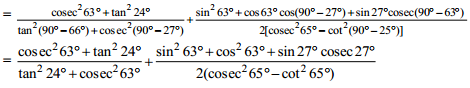{tex} = 1 + \frac{{1 + 1}}{{2(1)}} = 2{/tex}

OR
{tex}\sin \theta + \cos \theta = \sqrt 2 {/tex}
{tex} \Rightarrow {\left( {\sin \theta + \cos \theta } \right)^2} = {\left( {\sqrt 2 } \right)^2}{/tex}
{tex} \Rightarrow {\sin ^2}\theta + {\cos ^2}\theta + 2\sin \theta \cos \theta = 2{/tex}
{tex} \Rightarrow 1 + 2\sin \theta \cos \theta = 2{/tex}
{tex} \Rightarrow \sin \theta \cos \theta = \frac{1}{2}\,…(i){/tex}
{tex}we\,know,\,{\sin ^2}\theta + {\cos ^2}\theta = 1\,…(ii){/tex}
{tex}Dividing\,(ii)\,by\,(i)\,we\,get{/tex}
{tex}\frac{{{{\sin }^2}\theta + {{\cos }^2}\theta }}{{\sin \theta \cos \theta }} = \frac{1}{{1/2}}{/tex}
{tex} \Rightarrow \tan \theta + \cot \theta = 2{/tex}

20. In given figure ABPC is a quadrant of a circle of radius 14 cm and a semicircle is drawn with BC as diameter. Find the area of the shaded region.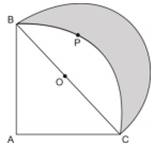Ans. We know, AC = r
{tex}In\,\Delta ACB,\,B{C^2} = A{C^2} + A{B^2}{/tex}
{tex} \Rightarrow BC = AC\sqrt 2 \left( {\because AB = AC} \right){/tex}
{tex} \Rightarrow BC = r\sqrt 2 {/tex}
Required area = {tex}ar\left( {\Delta ACB} \right){/tex} + ar(semicircle on BC as diameter) –ar(quadrant

ABPC
{tex} = \frac{1}{2} \times r \times r + \frac{1}{2} \times \pi \times {\left( {\frac{{r\sqrt 2 }}{2}} \right)^2} – \frac{1}{4}\pi {r^2}{/tex}
{tex} = \frac{{{r^2}}}{2} + \frac{{\pi {r^2}}}{4} – \frac{{\pi {r^2}}}{4}{/tex}
{tex} = \frac{{{r^2}}}{2} = \frac{{196}}{2}c{m^2} = 98\,c{m^2}{/tex}

21. Water in a canal, 6 m wide and 1.5 m deep, is flowing with a speed of 10 km/h. How much area will it irrigate in 30 minutes, if 8 cm of standing water is needed?
OR
A cone of maximum size is carved out from a cube of edge 14 cm. Find the surface area of the remaining solid after the cone is carved out.

Ans. Let the area that can be irrigated in 30 minute be A {tex}{m^2}.{/tex}
Water flowing in canal in 30 minutes {tex} = \left( {10,000 \times \frac{1}{2}} \right)m = 5000\,m{/tex}
Volume of water flowing out in 30 minutes {tex} = \left( {5000 \times 6 \times 1.5} \right)\,{m^3} = 45000\,{m^3}\,…(i){/tex}
Volume of water required to irrigate the field {tex} = A \times \frac{8}{{100}}\,{m^3}\,…(ii){/tex}
Equating (i) and (ii), we get
{tex}A \times \frac{8}{{100}} = 45000{/tex}
{tex}A = 562500\,{m^2}{/tex}

Or
{tex}l = \sqrt {{7^2} + {{14}^2}} = 7\sqrt 5 {/tex}
Surface area of remaining solid{tex} = 6{l^2} – \pi {r^2} + \pi rl,{/tex} where r and l are the radius and slant height of the cone.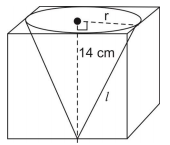{tex} = 6 \times 14 \times 4 – \frac{{22}}{7} \times 7 \times 7 + \frac{{22}}{7} \times 7 \times 7\sqrt 5 {/tex}
{tex} = 1176 – 154 + 154\sqrt 5 {/tex}
{tex} = \left( {1022 + 154\sqrt 5 } \right)c{m^2}{/tex}
22. Find the mode of the following distribution of marks obtained by the students in an examination:

 Marks obtained 0-20 20-40 40-60 60-80 80-100 Number of students 15 18 21 29 17

Given the mean of the above distribution is 53, using empirical relationship estimate the value of its median.

Ans. {tex}Mode = \ell + \left( {\frac{{{f_2} – {f_0}}}{{2{f_2} – {f_0} – {f_2}}}} \right) \times h{/tex}
{tex} = 60 + \left( {\frac{{29 – 21}}{{58 – 21 – 17}}} \right) \times 20 = 68{/tex}
So, the mode marks is 68.
Empirical relationship between the three measures of central tendencies is:
{tex}3\,Median = Mode + 2\,Mean{/tex}
{tex}3\,Median = 68 + 2 \times 53{/tex}{tex}Median = 58\,marks{/tex}

### Section D Question numbers 23 to 30 carry 4 marks each.

23. A train travelling at a uniform speed for 360 km would have taken 48 minutes less to travel the same distance if its speed were 5 km/hour more. Find the original speed of the train.
OR
Check whether the equation {tex}5{x^2}-6x-\;2 = 0{/tex} has real roots and if it has, find them by the method of completing the square. Also verify that roots obtained satisfy the given equation.

Ans. Let original speed of the train be x km/h.
Time taken at original speed {tex} = \frac{{360}}{x}\,hours{/tex}
Time taken at increased speed{tex} = \frac{{360}}{{x + 5}}\,hours{/tex}
Now, {tex}\frac{{360}}{x} – \frac{{360}}{{x + 5}} = \frac{{48}}{{60}}{/tex}
{tex} \Rightarrow 360\left[ {\frac{1}{x} – \frac{1}{{x + 5}}} \right] = \frac{4}{5}{/tex}
{tex} \Rightarrow {x^2} + 5x – 2250 = 0{/tex}
{tex} \Rightarrow x = 45\,or\, – 50{/tex}{tex}(as\,speed\,cannot\,be\,negative){/tex}
{tex} \Rightarrow x = 45\,km/h{/tex}
OR
Discriminant {tex} = {b^2} – 4ac = 36 – 4 \times 5 \times ( – 2) = 76 > 0{/tex}
So, the given equation has two distinct real roots
{tex}5{x^2} – 6x – 2 = 0{/tex}

Multiplying both sides by 5.
{tex}{(5x)^2} – 2 \times (5x) \times 3 = 10{/tex}
{tex} \Rightarrow {(5x)^2} – 2 \times (5x) \times 3 + {3^2} = 10 + {3^2}{/tex}
{tex} \Rightarrow {(5x – 3)^2} = 19{/tex}
{tex} \Rightarrow 5x – 3 = \pm \sqrt {19} {/tex}
{tex} \Rightarrow x = \frac{{3 \pm \sqrt {19} }}{5}{/tex}
Verification: {tex}5{\left( {\frac{{3 + \sqrt {19} }}{5}} \right)^2} – 6\left( {\frac{{3 + \sqrt {19} }}{5}} \right) – 2{/tex}{tex} = \frac{{9 + 6\sqrt {19} + 19}}{5} – \frac{{18 + 6\sqrt {19} }}{5} – \frac{{10}}{5} = 0{/tex}
Similarly, {tex}5{\left( {\frac{{3 – \sqrt {19} }}{5}} \right)^2} – 6\left( {\frac{{3 – \sqrt {19} }}{5}} \right) – 2 = 0{/tex}
24. An AP consists of 37 terms. The sum of the three middle most terms is 225 and the sum of the last three terms is 429. Find the AP.

Ans. Let the three middle most terms of the AP be a – d, a, a + d.
We have, (a – d) + a + (a + d) = 225
{tex} \Rightarrow 3a = 225 \Rightarrow a = 75{/tex}
Now, the AP is
a – 18d,…,a – 2d, a – d, a, a + d, a + 2d,…, a + 18d

Sum of last three terms:
{tex}(a + 18d) + (a + 17d) + (a + 16d) = 429{/tex}
{tex} \Rightarrow 3a + 51d = 429 \Rightarrow a + 17d = 143{/tex}
{tex} \Rightarrow 75 + 17d = 143 \Rightarrow d = 4{/tex}
Now, first term {tex} = a – 18d = 75 – 18(4) = 3{/tex}
{tex}\therefore {/tex}The AP is 3, 7, 11, …, 147.

25. Show that in a right triangle, the square of the hypotenuse is equal to the sum of the squares of the other two sides.
OR
Prove that the ratio of the areas of two similar triangles is equal to the ratio of the squares of their corresponding sides.

Ans. Given: A right triangle ABC right angled at B.
To prove: {tex}A{C^2} = A{B^2} + B{C^2}{/tex}
Construction: Draw {tex}BD \bot AC{/tex}
{tex}\angle ADB = \angle ABC(each\,90^\circ ){/tex}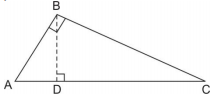Now, {tex}\frac{{AD}}{{AB}} = \frac{{AB}}{{AC}}{/tex}(corresponding sides are proportional)
{tex} \Rightarrow A{B^2} = AD \times AC\,…(i){/tex}
Similarly {tex}\Delta BDC \sim \Delta ABC{/tex}
{tex} \Rightarrow B{C^2} = CD \times AC\,…(ii){/tex}

{tex}A{B^2} + B{C^2} = AD \times AC + CD \times AC{/tex}
{tex} \Rightarrow A{B^2} + B{C^2} = AC \times (AD + CD){/tex}
{tex} \Rightarrow A{B^2} + B{C^2} = A{C^2},\,Hence\,proved.{/tex}
OR
Given: {tex}\Delta ABC \sim \Delta PQR{/tex}
To prove: {tex}\frac{{ar(\Delta ABC)}}{{ar(\Delta PQR)}} = {\left( {\frac{{AB}}{{PQ}}} \right)^2} = {\left( {\frac{{BC}}{{QR}}} \right)^2} = {\left( {\frac{{CA}}{{RP}}} \right)^2}{/tex}
Construction: Draw {tex}AM \bot BC,\,PN \bot QR{/tex}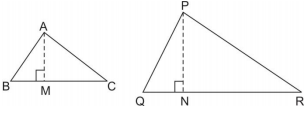{tex}\frac{{ar(\Delta ABC)}}{{ar(\Delta PQR)}} = \frac{{\frac{1}{2} \times BC \times AM}}{{\frac{1}{2} \times QR \times PN}}{/tex}{tex} = \frac{{BC}}{{QR}} \times \frac{{AM}}{{PN}}\,…(i){/tex}
In {tex}\Delta ABM\,and\,\Delta PQN{/tex}
{tex}\angle B = \angle Q(\because \Delta ABC \sim \Delta PQR){/tex}
{tex}\angle M = \angle N(each\,90^\circ ){/tex}
{tex}\Delta ABM \sim \Delta PQN{/tex} {tex}(AA\,similarity\,criterion){/tex}
Therefore, {tex}\frac{{AM}}{{PN}} = \frac{{AB}}{{PQ}}\,…(ii){/tex}
But {tex}\frac{{AB}}{{PQ}} = \frac{{BC}}{{QR}} = \frac{{CA}}{{RP}}\left( {\Delta ABC \sim \Delta PQR} \right)\,…(iii){/tex}

Hence, {tex}\frac{{ar(\Delta ABC)}}{{ar(\Delta PQR)}} = \frac{{BC}}{{QR}} \times \frac{{AM}}{{PN}}\,from\,(i){/tex}
{tex} = \frac{{AB}}{{PQ}} \times \frac{{AB}}{{PQ}}[from\,(ii)\,and\,(iii)]{/tex}
{tex} = {\left( {\frac{{AB}}{{PQ}}} \right)^2}{/tex}
{tex}\frac{{ar(\Delta ABC)}}{{ar(\Delta PQR)}} = {\left( {\frac{{AB}}{{PQ}}} \right)^2} = {\left( {\frac{{BC}}{{QR}}} \right)^2}{/tex}{tex} = {\left( {\frac{{CA}}{{RP}}} \right)^2}{\text{Using}}\,(iii){/tex}
26. Draw a triangle ABC with side {tex}BC = 7cm,{\text{ }}\angle B = 45^\circ ,{\text{ }}\angle A = 105^\circ .{/tex} Then, construct a triangle whose sides are {tex}\frac{4}{3}{/tex} times the corresponding sides of {tex}\Delta ABC.{/tex}

Ans. Draw {tex}\Delta ABC{/tex} in which {tex}BC = 7\,cm,\,\angle B = 45^\circ ,\,\angle A = 105^\circ {/tex} and hence {tex}\angle C = 30^\circ .{/tex} Construction of similar triangle {tex}A’BC'{/tex} as shown below: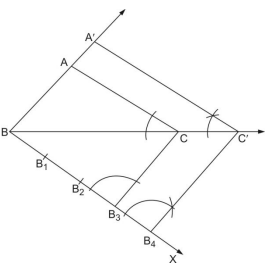27. Prove that {tex}\frac{{\cos \theta – \sin \theta + 1}}{{\cos \theta + \sin \theta – 1}} = \cos ec\theta + \cot \theta {/tex}

Ans. {tex}LHS = \frac{{\cos \theta – \sin \theta + 1}}{{\cos \theta + \sin \theta – 1}}{/tex}
{tex} = \frac{{\cos \theta – \sin \theta + 1}}{{\cos \theta + \sin \theta – 1}} \times \frac{{\cos \theta + \sin \theta + 1}}{{\cos \theta + \sin \theta + 1}}{/tex}
{tex} = \frac{{{{(\cos \theta + 1)}^2} – {{\sin }^2}\theta }}{{{{(\cos \theta + \sin \theta )}^2} – {1^2}}}{/tex}
{tex} = \frac{{{{\cos }^2}\theta + 1 + 2\cos \theta – {{\sin }^2}\theta }}{{{{\cos }^2}\theta + {{\sin }^2}\theta + 2\sin \theta \cos \theta – 1}}{/tex}
{tex} = \frac{{2{{\cos }^2}\theta + 2\cos \theta }}{{2\sin \theta \cos \theta }}{/tex}
{tex} = \frac{{2\cos \theta (\cos \theta + 1)}}{{2\sin \theta \cos \theta }}{/tex}
{tex} = \frac{{\cos \theta + 1}}{{\sin \theta }} = {\text{cosec}}\theta {\text{ + cot}}\theta {\text{ = RHS}}{/tex}

28. The angles of depression of the top and bottom of a building 50 metres high as observed from the top of a tower are {tex}30^\circ {\text{ }}and{\text{ }}60^\circ ,{/tex} respectively. Find the height of the tower and also the horizontal distance between the building and the tower.

Ans. {tex}In\,\Delta BTP \Rightarrow \tan \,30^\circ = \frac{{TP}}{{BP}}{/tex}
{tex} \Rightarrow \frac{1}{{\sqrt 3 }} = \frac{{TP}}{{BP}}{/tex}
{tex}BP = TP\sqrt 3 \,…(i){/tex}
{tex}In\,\Delta GTR,{/tex}
{tex}\tan \,60^\circ = \frac{{TR}}{{GR}} \Rightarrow \sqrt 3 = \frac{{TR}}{{GR}}{/tex}{tex} \Rightarrow GR = \frac{{TR}}{{\sqrt 3 }}\,..(ii){/tex}
Now, {tex}TP\sqrt 3 = \frac{{TR}}{{\sqrt 3 }}(as\,BP = GR){/tex}
{tex} \Rightarrow 3TP = TP + PR{/tex}
{tex} \Rightarrow 2TP = BG \Rightarrow TP = \frac{{50}}{2}m = 25m{/tex}
Now, {tex}TR = TP + PR = (25 + 50)m{/tex}
Height of tower = TR = 75 m
Distance between building and tower {tex} = GR = \frac{{TR}}{{\sqrt 3 }}{/tex}
{tex} \Rightarrow GR = \frac{{75}}{{\sqrt 3 }}m = 25\sqrt 3 \,m{/tex}
29. Two dairy owners A and B sell flavoured milk filled to capacity in mugs of negligible thickness, which are cylindrical in shape with a raised hemispherical bottom. The mugs are 14 cm high and have diameter of 7 cm as shown in given figure.

Both A and B sell flavoured milk at the rate of {tex}Rs.\,80{/tex} per litre. The dairy owner A uses the formula {tex}\pi {r^2}h{/tex} to find the volume of milk in the mug and charges {tex}Rs.\,\;43.12{/tex} for it. The dairy owner B is of the view that the price of actual quantity of milk should be charged. What according to him should be the price of one mug of milk? Which value is exhibited by the dairy owner B? {tex}\left( {use\,\pi = \frac{{22}}{7}} \right){/tex}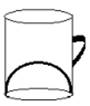Ans. Capacity of mug (actual quantity of milk){tex} = \pi {r^2}h – \frac{2}{3}\pi {r^3}{/tex}
{tex} = \pi {r^2}\left( {h – \frac{2}{3}r} \right){/tex}
{tex} = \frac{{22}}{7} \times \frac{7}{2} \times \frac{7}{2} \times \left( {14 – \frac{2}{3} \times \frac{7}{2}} \right){/tex}
{tex} = \frac{{2695}}{6}c{m^3}{/tex}
Amount dairy owner B should charge for one mug of milk {tex} = \frac{{2695}}{6} \times \frac{{80}}{{1000}} = Rs.\,35.93{/tex}
Value exhibited by dairy owner B: honesty (or any similar value)

30. The following distribution shows the daily pocket allowance of children of a locality. The mean pocket allowance is {tex}Rs.\,18.{/tex} Find the missing frequency k.

 Daily pocket allowance (in Rs.) 11-13 13-15 15-17 17-19 19-21 21-23 23-25 Number of children 3 6 9 13 k 5 4

OR
The following frequency distribution shows the distance (in metres) thrown by 68 students in a Javelin throw competition.

 Distance (in m) 0-10 10-20 20-30 30-40 40-50 50-60 60-70 Number of students 4 5 13 20 14 8 4

Draw a less than type Ogive for the given data and find the median distance thrown using this curve.

Ans.

 Daily pocket allowance (in Rs.) Number of children {tex}\left( {{f_i}} \right){/tex} Mid-point {tex}({x_i}){/tex} {tex}{u_i} = \frac{{{x_i} – 18}}{2}{/tex} {tex}{f_i}{u_i}{/tex} 11 – 13 3 12 -3 -9 13 – 15 6 14 -2 -12 15 – 17 9 16 -1 -9 17 – 19 13 18 0 0 19 – 21 K 20 1 k 21 – 23 5 22 2 10 23 – 25 4 24 3 12 {tex}\sum {f_i} = 40 + k{/tex} {tex}\sum {f_i}{u_i} = k – 8{/tex}

{tex}Mean = \bar x = a + h\left( {\frac{{\sum {f_i}{u_i}}}{{\sum {f_i}}}} \right){/tex}
{tex}18 = 18 + 2\left( {\frac{{k – 8}}{{40 + k}}} \right){/tex}
{tex} \Rightarrow k = 8{/tex}
OR

 Less than Number of Students 10 4 20 9 30 22 40 42 50 56 60 64 70 68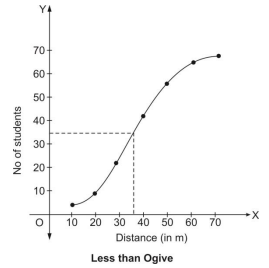Median distance is value of x that corresponds to Cumulative frequency {tex}\frac{N}{2} = \frac{{68}}{2} = 34{/tex}
Therefore, Median distance = 36 m

This is only initial part of the whole sample paper. Download Complete set of sample paper for class 10 Maths

## Sample paper for class 10 Maths

It is sample paper for class 10 Maths. However, myCBSEguide provides the best sample papers for all the subjects. There are number of sample papers which you can download from myCBSEguide website.

## Sample paper for class 10 all subjects

To download complete sample paper for class 10 Maths , Science, Social Science, Hindi and English; do check myCBSEguide app or website. myCBSEguide provides sample papers with solution, test papers for chapter-wise practice, NCERT solutions, NCERT Exemplar solutions, quick revision notes for ready reference, CBSE guess papers and CBSE important question papers. Sample Paper all are made available through the best app for CBSE students and myCBSEguide website.### Test Generator

Create question paper PDF and online tests with your own name & logo in minutes.### myCBSEguide

Question Bank, Mock Tests, Exam Papers, NCERT Solutions, Sample Papers, Notes

### 6 thoughts on “Sample paper for class 10 Maths 2018-19”

1. nice syllabus

2. nice

3. tough but nice and imp

4. Tough this time

5. nice

6. nice questians